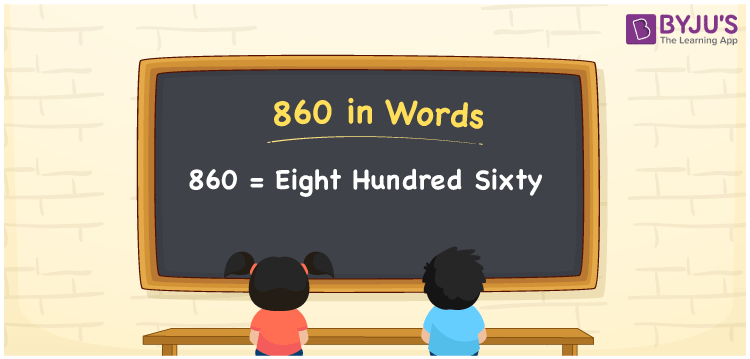# 860 in Words

860 in words can be written as Eight Hundred Sixty. If you have to write an essay containing 860 words, then you can say that “I have to write an essay containing Eight Hundred Sixty words”. The numbers in words concept has various applications in our lives. Therefore, 860 can be read as “Eight Hundred Sixty” in words.

 860 in words Eight Hundred Sixty Eight Hundred Sixty in Numbers 860

## 860 in English Words## How to Write 860 in Words?

There are three digits present in the number 860. Let us create a place value chart for the number 860 in this section.

 Ten Thousands Thousands Hundreds Tens Ones 1 4 5 6 0

860 in expanded form is:

8 × Hundred + 6 × Ten + 0 × One

= 8 × 100 + 6 × 10 + 0 × 1

= 800 + 60

= 860

= Eight Hundred Sixty

Therefore, 860 in words is written as Eight Hundred Sixty.

860 is a natural number that precedes 861 and succeeds 859.

860 in words – Eight Hundred Sixty

Is 860 an odd number? – No

Is 860 an even number? – Yes

Is 860 a perfect square number? – No

Is 860 a perfect cube number? – No

Is 860 a prime number? – No

Is 860 a composite number? – Yes

## Frequently Asked Questions on 860 in Words

Q1

### Write 860 in words.

860 can be written in words as “Eight Hundred Sixty”.
Q2

### How do you write Eight Hundred Sixty in numbers?

Eight Hundred Sixty can be written in numbers as 860.
Q3

### Is 860 an even number?

Yes, 860 is an even number as it is completely divisible by 2.